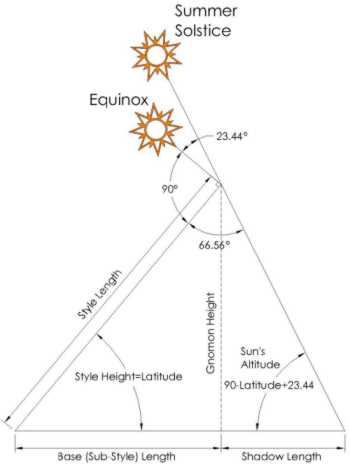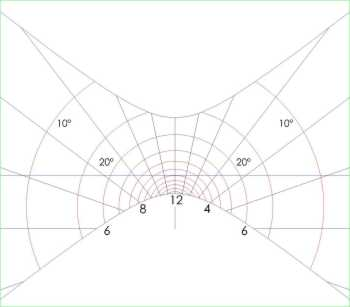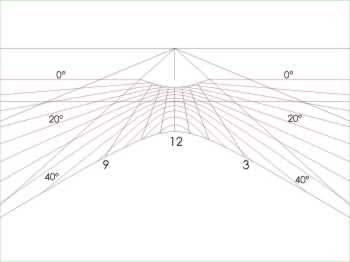The Sundial Primer created by Carl SabanskiThe Sundial Primer Index
 Altitude Lines Altitude (of the sun) (a, ALT): the angular distance of the centre of the sun's disk above the observer's horizon (negative numbers indicate that the sun is below the horizon). It is measured along the principal plane to the sun's centre, and is the complement of the zenith distance. It is part of the horizontal co-ordinate system.Horizon: the line of intersection between the sky and the Earth. For normal astronomical purposes, the observer's horizon is taken to be the great circle on the celestial sphere on which every point is 90° from the observer's zenith. The observed horizon (accounting for the curve of the earth and the height of the observer above its surface, but excluding refraction) is below the astronomical horizon by an angle called the dip. This can have a significant affect on the times of sunrise/sunset.Zenith: the point on the celestial sphere vertically above the observer. Altitude lines trace the angular distance of the sun above the horizon. Determining the altitude lines for a horizontal sundial is not difficult. Any given altitude will be represented by a circle centered at a point on the dial plate directly below the nodus. In Figure 1 this is the point below the tip of the style. The radius of an altitude line is:Radius = Gnomon Height / tan (Sun's Altitude) The radius is the "Shadow Length" as shown in Figure 1.Figure 1: Horizontal Sundial Altitude Lines (CAD) The maximum altitude that the sun can reach at any given latitude is determined as follows:Maximum Altitude = 90° + 23.44° - Latitude Maximum Altitude = 113.44° - LatitudeThe following figures show various dials with the altitude lines included for a common nodus height. The layout of the altitude lines will be affected by the height of the nodus.Figure 2: Altitude Lines - Horizontal Sundial (ZW2000/CAD)As can be seen, the altitude lines for a horizontal dial are concentric circles. They are shown at 5° intervals from 10° to 60°. From this figure you can see how the method of equal altitudes can be used to determine the direction of true north. This technique is discussed on the page "Finding True North".Figure 3: Altitude Lines - Vertical Direct South Sundial (ZW2000/CAD) For a vertical sundial the altitude lines are quite different. They are shown at 5° intervals from 0° to 60°.